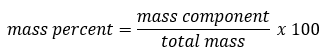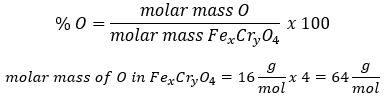# Problem: A chromium-containing compound has the formula FexCryO4 and is 28.59% oxygen by mass.Find x.

###### FREE Expert Solution

Recall the formula for mass percent:Mass percent O = 28.59%###### Problem Details

A chromium-containing compound has the formula FexCryO4 and is 28.59% oxygen by mass.

Find x.